## 介绍

John Holland 和他的合作者在 20 世纪 60 年代和 70 年代，基于达尔文的自然选择理论的生物进化模型，开发了遗传算法（Genetic algorithm，GA）[11, 4] 。Holland 可能是第一个在自适应和人工系统研究中使用交叉、重组、变异和选择的人。作为解决问题的策略，这些遗传算子构成遗传算法的基本部分。从那以后，遗传算法的许多变体被开发出来，并且被应用于各种各样的优化问题，从图着色到模式识别，从离散系统（如旅行商问题）到连续系统（如航空航天工程领域，翼型的有效设计），从金融市场到多目标工程优化。

## 遗传算法

1. 对目标或代价（cost）函数进行编码;
2. 定义适应度函数或选择标准;
3. 创建种群、个体;
4. 通过评估种群中所有个体的适应度来完成进化周期（evolution cycle）或迭代，通过进行交叉和变异，适应性比例繁殖等来创建一个新种群，并替换旧种群并重新迭代新的种群;
5. 解码结果以获得问题的解。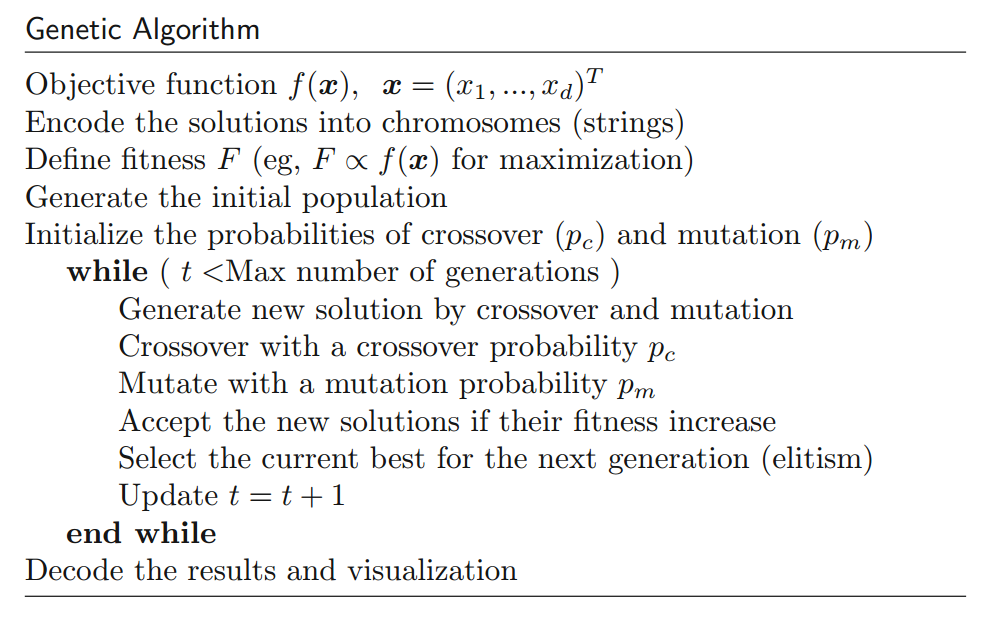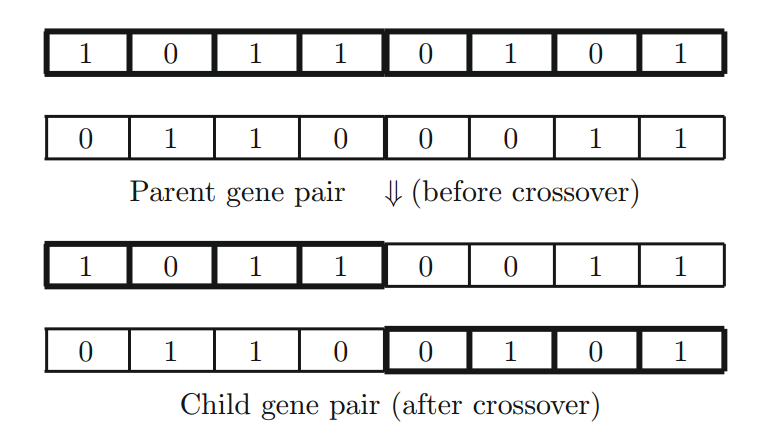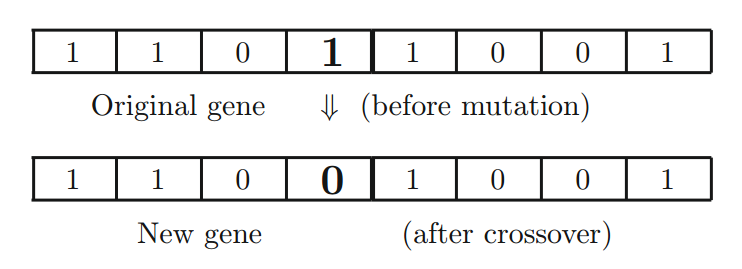## 遗传算子的作用

• 交叉（Crossover）。将染色体或解中的一部分解与另一部分解交换。主要作用是提供解的混合和子空间中的收敛。
• 变异（Mutation）。解的一部分随机变化，这增加了种群的多样性，并提供逃离局部最优化的机制。
• 选择或精英主义（Selection of the fittest, or elitism）。高质量解将传给下一代，这通常是通过某种形式的选择最佳解来进行的。

## 参数的选择

$$F(x_i) = \frac{f(\xi_i)}{\sum_{i=1}^n f(\xi_i)} \tag 1 其中 \xi_i 是个体 i 的表型值（phenotypic value）、n 是群体规模。 适应度函数的适当形式将确保高效地选择具有较高适应度的解。适应度函数差可能导致解不正确或毫无意义。 另一个重要问题是各种参数的选择。交叉概率 p_c 通常非常高，通常在 0.7 〜 1.0 的范围内。另一方面，变异概率 p_m 通常很小（通常为 0.001 〜 0.05）。如果 p_c 太小，那么交叉就会稀疏地发生，这对于进化是不利的。如果变异概率太高，即使最佳解即将到来，解仍然可能“跳来跳去”。 选择最佳解的适当标准也很重要。如何选择当前的种群，以便保留较高适应度的优秀个体并传递给下一代，这是一个尚未完全解答的问题。选择往往是与某些精英主义联系在一起进行的。最基本的精英主义是选择最合适的个体(每一代)，其特征将被遗传算子传递到下一代。这确保更快地到达最佳解。 其他问题包括多变异位点和各种群体规模的使用。单位点的变异并不十分有效；多位点的变异将增加进化效率。然而，太多的变异会使系统很难收敛，甚至会使系统陷入错误的解。在真实的生态系统中，如果在高选择压力下变异率过高，整个种群可能会灭绝。 此外，正确种群规模 n 的选择也非常重要。如果种群规模太小，没有足够的进化发展，整个种群就有可能灭绝。在现实世界中，对于种群规模较少的物种而言，生态学理论认为这种物种确实存在灭绝危险。即使系统继续运行，仍然存在过早收敛的危险。在在一个小群体中，如果一个明显更适应（fit）的个体出现得太早，它可能会繁殖出足够多的后代，使他们压倒整个（小）群体。这最终将使系统达到局部最优（而不是全局最优）。另一方面，如果群体过多，则需要对目标函数进行更多的评估，这将花费大量计算时间。研究和实证观察表明，n = 40 到 200 的种群规模适用于大多数问题。 此外，对于更复杂的问题，可以使用各种 GA 变体来执行特定的任务。文献中可以找到自适应遗传算法和混合遗传算法等变体。 使用所描述的基本过程，我们可以用任何编程语言实现遗传算法。从实现的角度来看，有两种使用编码字符串的主要方式。我们可以对群体中的每个个体使用单独的字符串（或多个字符串），也可以对所有个体或变量使用一个长字符串，如下图所示。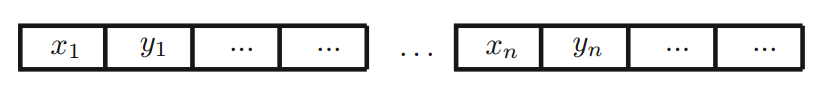著名的 Easom 函数 $$f(x) = - cos(x) e ^{- (x - \pi)^2}, \quad x \in [-10, 10] \tag 2$$

$x_* = \pi$ 处具有全局最大值 $f_{max} = 1$。典型运行的输出如下图所示，其中上图显示最佳估计 $x_* \to \pi$ 的变化，下图显示适应度函数的变化。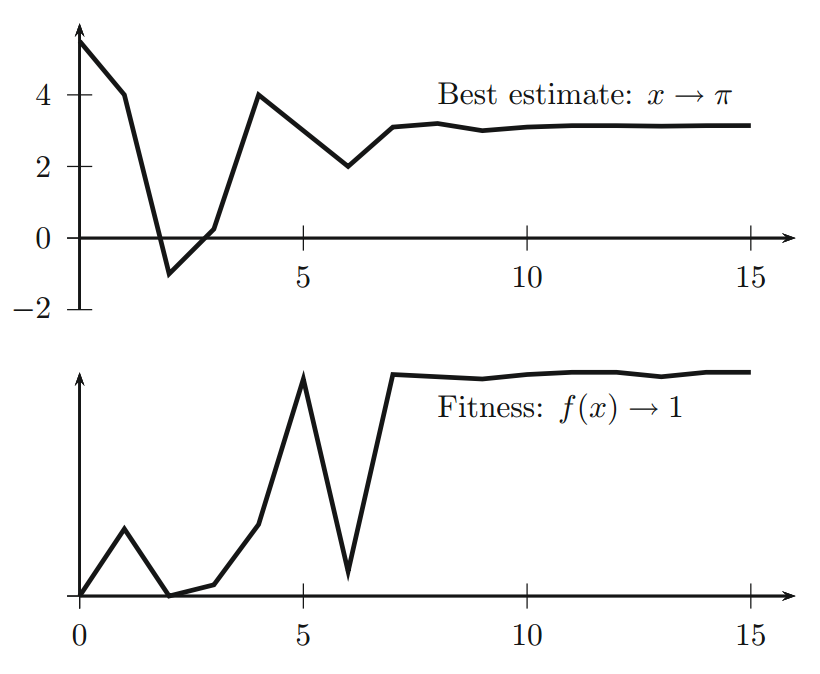## 模式定理

Holland 的适应度比例选择算子可以定义为个体 $h$ 对模式 $H$ 进行抽样的概率 $p$

$$p (h \in H) = \frac{k_{H,t} \bar f(H, t)}{n \bar f(t)} \tag 3 其中 n 是种群规模、\bar f(t) 是第 t 代的平均适应度， $$\bar f(t) = \frac 1n \sum_{i=1}^n f_i \tag 4$$

$$E[k_{H, t+1}] = n \; p(h \in H) = \frac{k_{H,t} \bar f(H, t)}{\bar f(t)} \tag 5 著名的模式定理可以表述如下： $$E[k_{H, t+1}] \ge k_{H, t} \frac{\bar f(H, t)}{\bar f(t)} \bigl [ 1 - p_c \frac{\delta (H)}{m - 1}\bigr] (1 - p_m)^{o(H)} \tag 6$$

$$E[k_{H, t+1}] \ge k_{H, t} \frac{\bar f(H, t)}{\bar f(t)} \Bigl [ 1 - \frac{\delta (H)}{(m - 1)} p_c - o(H) p_m \Bigr] \tag 7 这个定理意味着具有高于平均水平的低阶图更可能在人群中存活。这就是所谓的Goldberg的积木式假设或猜想。简而言之，低阶短模式通常被称为构建模块，这一假设表明，通过重组或交叉，高度变异，短期和低阶积木块逐步形成更好的解决方案。进一步的数学分析和实际意义仍然是一个积极的研究课题。 这个定理意味着，具有高于平均概率的低阶模式更有可能在群体中生存。这就是所谓的 Goldberg 积木块假设/猜想（building-block hypothesis） 。简而言之，低阶、短长度的模式通常被称为积木块。这一假设表明，更好的解会通过积木块的重组或交叉以及高适应度变异，逐步形成。进一步的数学分析和实际意义仍然是一个活跃的研究课题。 尽管这个模式定理有重要结果，但它几乎没有关于遗传算法收敛速度的信息。因此，在本章其余部分中，我们简要介绍一些关于 GA 收敛的结果。 ## 收敛性分析 关于遗传算法收敛性的研究很多，关键结果可以在几十篇理论文献中找到 [3, 10, 12, 14-16]。然而，从介绍的角度来看，在本章其余部分，我们主要介绍 Louis 和 Rawlins 的结果  ，他们的结果为遗传算法的主要特征提供了一些很好的见解。 在我们继续之前，让我们介绍一下汉明距离（Hamming distance）的基本概念，它是两个等长的串之间的距离度量。实质上，汉明距离是两个字符串中相应字符不同的符号或位置的数量。换句话说，如果从一个字符串开始，那么需要更换为另一个字符串的替换或替换的最小数量。例如，theorymemory 之间的汉明距离是 3，因为只有前三个字符是不同的。12345671122117 之间的汉明距离是 5。 Louis 和 Rawlins 的主要成果可以总结如下。对于 n 个二进制字符串的集合，每个字符串的固定长度为 m 且有 n(n-1)/2 对样本。平均汉明距离可以用均值为 H_0、标准差为 \sigma_0 的正态分布来估计。即， $$H_0 = \frac m2, \quad \sigma_0 = \frac {\sqrt m}{2} \tag 8$$

$${n \choose k} p_j^k (1 - p_j) ^{(n - k)} \tag 9 原则上，可以估计等位基因出现的特定频率的概率，但数学分析是复杂的。对于当前目的，在 t 代等位基因频率取值 p 的概率 A(p, t) 可以近似为 $$A(p, t) = \frac {6 p_0 (1 - p_0)}{n} \bigl ( 1 - \frac 2n\bigr)^t \tag {10}$$

$$p(t, m) = \Bigl [ 1 - \frac{6 p_0 (1 - p_0)}{n} \bigl ( 1 - \frac 2n\bigr )^t \Bigr]^m \tag {11} 当目标函数是常数时，它为函数提供了一个上界。例如，对于 n = 40，具有 100 位的染色体的群体，在 100 代之后，我们有 t = 100m = 100n = 40 以及 p_0 = 0.5（二元）。初始的汉明平均距离是m/2。因此，前面的概率变成了 $$P(t, m) = \Bigl [ 1 - \frac{6 \times 0.5 (1 - 0.5)}{40} (1 - \frac{2}{40})^{100}\Bigl]^{100} \approx 0.978 \tag {12}$$

$$t(\xi) \le \biggl \lceil \frac{ln(1 - \xi)}{ ln \Bigl \{ 1 - min \bigl [ (1 - \mu)^{Ln}, \mu^{Ln} \bigr] \Bigr \} } \biggr \rceil \tag {13} 其中 \mu = 突变率、L = 字符串长度、n = 种群规模。这对于单目标优化是有效的。 对于多目标优化，也有一些有趣的结果。例如，Villalobos-Arias 等人提出一个元启发式算法的转移矩阵 P 有一个平稳分布 \pi $$\vert P_{ij}^k - \pi_j \vert \le (1 - \xi)^{k - 1}, \quad \forall i, j, \quad (k = 1, 2, ...) \tag {14}$$

 Aytug H, Bhattacharrya S, Koehler GJ. A Markov chain analysis of genetic algorithms with power of 2 cardinality alphabets. Euro J Operational Res 1996;96(1):195–201.

 Aytug H, Koehler GJ. New stopping criterion for genetic algorithms. Euro J Operational Res 2000;126(2):662–74.

 Eiben AE, Aarts EHL, Van Hee KM. Global convergence of genetic algorithm: a Markov chain analysis. In: Schwefel H-P, Männer R, editors. Parallel problem solving from nature. Berlin, Germany: Springer; 1991. p. 4–12.

 De Jong K, Analysis of the behavior of a class of genetic adaptive systems, Ph.D. thesis, University of Michigan, Ann Arbor, MI, USA; 1975.

 Fogel DB. Evolutionary computation: toward a new philosophy of machine intelligence. 3rd ed. Piscataway, NJ.: IEEE Press; 2006.

 Gale JS. Theoretical population genetics. London, UK: Unwin Hyman Ltd; 1990.

 Goldberg DE. Genetic algorithms in search, optimisation and machine learning. Reading, MA, USA: Addison-Wesley; 1989.

 Greenhalgh D, Marshal S. Convergence criteria for genetic algorithms. SIAM J Comput 2000;30(1):269–82.

 Gutjahr WJ. Convergence analysis of metaheuristics. Anna Inf Sys 2010;10(1):159–87.

 He J, Kang LS. On the convergence rates of genetic algorithms. Theor Comput Sci 1999;229(1):23–9.

 Holland J. Adaptation in natural and artiﬁcial systems. Ann Arbor, MI, USA: University of Michigan Press; 1975.

 Louis SJ, Rawlins GJE. Predicting convergence time for genetic algorithms. In: Whitley LD, editor. Foundations of genetic algorithms, vol. 2. Los Altos, CA, USA: Morgan Kaufman;1993. p. 141–61.

 Michalewicz Z. Genetic algorithms + data structures = evolution programs. SpringerVerlag; 1999.

 Rudolph G. Convergence analysis of canonical genetic algorithms. IEEE Trans Neural Netw 1994;5(1):96–101.

 Sharapov RR. Genetic algorithms: basic ideas, variants and analysis. In: Obinata G, Dutta A, editors. Vision systems: segmentation and pattern recognition. Vienna, Austria: I-Tech; June 2007.

 Suzuki J. A Markov chain analysis on simple genetic algorithms. IEEE Trans Sys Man Cybern 1995;25(4):655–9.

 Villalobos-Arias M, Coello CAC, Hernández-Lerma O. Asymptotic convergence of metaheuristics for multiobjective optimization problems. Soft Comput 2005;10(11):1001–5.